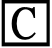### Home > PC > Chapter 10 > Lesson 10.2.3 > Problem10-107

10-107.

Challenge: Assuming no air resistance and a ball always thrown at $7$ feet above the ground with an initial velocity of $120$ ft/s, at what angle should the ball be released to go a maximum distance?This occurs when$y\left(t\right) = 0$.

Solve for $t$ (in terms of $θ$) if $−16t^2 + 120\left(\sin θ\right)t + 7 = 0$.

The answer for t is not pretty, but substitute it into the equation $x\left(t\right) = 120\left(\cos θ\right)t$.

You will need to graph this to determine when the distance is a maximum.